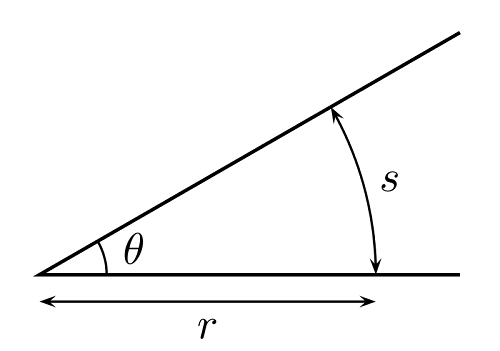## Types of Measurements in Surveying | Civil Engineering

#### Types of Measurements in Surveying

Surveying is the art of making suitable measurements in horizontal or vertical planes. This is one of the important subjects of civil engineering. Without taking a survey of the plot where the construction is to be carried out, the work cannot begin.

#### From the above definition, we conclude on two types of measurements in surveying. They are as follows:

1. Linear measurements
2. Angular measurements

Now we will go on with the discussion of each of these types of measurements along with their subtypes.

#### Linear measurements are further classified as follows:

Horizontal Distance

Vertical Distance

#### Horizontal Distance

A horizontal distance is measured in horizontal plane if a distance is measured along a slope, it is reduced to its horizontal equivalent.Horizontal Angle

#### Vertical Distance

A vertical distance is measured along the direction of gravity at that point. The vertical distance are measured to determine difference in elevations in various points.

Read more

## Introduction to Surveying | Civil Engineering

#### Surveying

Surveying is the art of making suitable measurements in horizontal or vertical planes. This is one of the important subjects of civil engineering. Without taking a survey of the plot where the construction is to be carried out, the work cannot begin.Surveying Chart

#### Levelling

Levelling is the art of representing relative positions in the vertical plane of different points on the earth’s surface.

It helps in determining the areas that are to be levelled to achieve a certain slope.

#### Principles of Surveying

All the surveys that are conducted are based on two fundamental principles. They are as follows:

1. Working from whole to part
2. Fixing a point with reference to two fixed points

#### Working from whole to part

In order to localize errors and prevent their accumulation, a set of control points is always established with great precision first for the whole area to be surveyed.

Later on, details or filled in between these control points to a relatively smaller precision.

This fundamental work principle is known as “Working from whole to part”.

#### Fixing a point with reference to two fixed points

Survey stations are fixed by atleast two measurements, either both linear or angular measurements or linear and angular measurements from two control points.

Read more